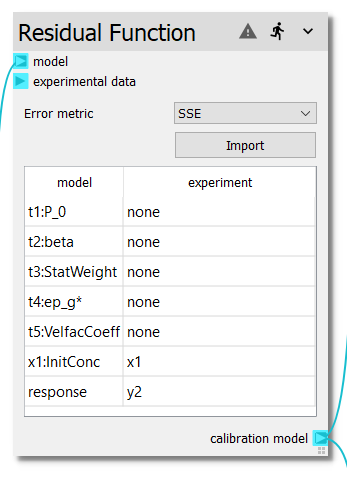# Residual Function¶

The Residual Function node combines a surrogate model from the Response Surface with experimental data, resulting in a new calibration model where the response is a user selectable error function. The model can then be used with other nodes such as the :ref: sma-optimizer node and the Sensitivity Analysis node. See the Ex. 5: Deterministic Calibration example for a demonstration of this nodes use.The following error metrics can be used as the response:

The experimental data can either be provided through the experimental data terminal or a CSV file can be imported by clicking the Import button. Once the node has an input model and experimental data, it needs to be run to build the table. Once the table has been populated, the user can map experimental values to the model values. Any values marked as none will be input parameters to the resulting calibration model (i.e. if the calibration model is connected to a :ref: sma-optimizer, the values marked with none will be varied by the :ref: sma-optimizer).

Internally, for every calibration model evaluation, every experimental sample is evaluated at those input values. The resulting evaluations are then used to calculate the response value (i.e. one of the error metrics above.)